# logic gate

## Gate universality

NAND and NOR gates possess a special property: they are universal. That is, given enough gates, either type of gate is able to mimic the operation of any other gate type. For example, it is possible to build a circuit exhibiting the OR function using three interconnected NAND gates. The ability for a single gate type to be able to mimic any other gate type is one enjoyed only by the NAND and the NOR. In fact, digital control systems have been designed around nothing but either NAND or NOR gates, all the necessary logic functions being derived from collections of interconnected NANDs or NORs.

As proof of this property, this section will be divided into subsections showing how all the basic gate types may be formed using only NANDs or only NORs.

### Constructing the NOT functionAs you can see, there are two ways to use a NAND gate as an inverter, and two ways to use a NOR gate as an inverter. Either method works, although connecting TTL inputs together increases the amount of current loading to the driving gate. For CMOS gates, common input terminals decreases the switching speed of the gate due to increased input capacitance.

Inverters are the fundamental tool for transforming one type of logic function into another, and so there will be many inverters shown in the illustrations to follow. In those diagrams, I will only show one method of inversion, and that will be where the unused NAND gate input is connected to +V (either Vcc or Vdd, depending on whether the circuit is TTL or CMOS) and where the unused input for the NOR gate is connected to ground. Bear in mind that the other inversion method (connecting both NAND or NOR inputs together) works just as well from a logical (1’s and 0’s) point of view, but is undesirable from the practical perspectives of increased current loading for TTL and increased input capacitance for CMOS.

### Constructing the “buffer” function

Being that it is quite easy to employ NAND and NOR gates to perform the inverter (NOT) function, it stands to reason that two such stages of gates will result in a buffer function, where the output is the same logical state as the input.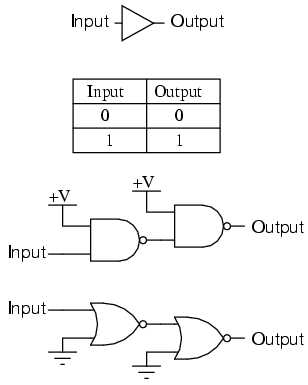### Constructing the AND function

To make the AND function from NAND gates, all that is needed is an inverter (NOT) stage on the output of a NAND gate. This extra inversion “cancels out” the first N in NAND, leaving the AND function. It takes a little more work to wrestle the same functionality out of NOR gates, but it can be done by inverting (“NOT”) all of the inputs to a NOR gate.### Constructing the NAND function

It would be pointless to show you how to “construct” the NAND function using a NAND gate, since there is nothing to do. To make a NOR gate perform the NAND function, we must invert all inputs to the NOR gate as well as the NOR gate’s output. For a two-input gate, this requires three more NOR gates connected as inverters.### Constructing the OR function

Inverting the output of a NOR gate (with another NOR gate connected as an inverter) results in the OR function. The NAND gate, on the other hand, requires inversion of all inputs to mimic the OR function, just as we needed to invert all inputs of a NOR gate to obtain the AND function. Remember that inversion of all inputs to a gate results in changing that gate’s essential function from AND to OR (or vice versa), plus an inverted output. Thus, with all inputs inverted, a NAND behaves as an OR, a NOR behaves as an AND, an AND behaves as a NOR, and an OR behaves as a NAND. In Boolean algebra, this transformation is referred to as DeMorgan’s Theorem, covered in more detail in a later chapter of this book.### Constructing the NOR function

Much the same as the procedure for making a NOR gate behave as a NAND, we must invert all inputs and the output to make a NAND gate function as a NOR.• REVIEW:
• NAND and NOR gates are universal: that is, they have the ability to mimic any type of gate, if interconnected in sufficient numbers.

## Logic signal voltage levels

Logic gate circuits are designed to input and output only two types of signals: “high” (1) and “low” (0), as represented by a variable voltage: full power supply voltage for a “high” state and zero voltage for a “low” state. In a perfect world, all logic circuit signals would exist at these extreme voltage limits, and never deviate from them (i.e., less than full voltage for a “high,” or more than zero voltage for a “low”). However, in reality, logic signal voltage levels rarely attain these perfect limits due to stray voltage drops in the transistor circuitry, and so we must understand the signal level limitations of gate circuits as they try to interpret signal voltages lying somewhere between full supply voltage and zero.

TTL gates operate on a nominal power supply voltage of 5 volts, +/- 0.25 volts. Ideally, a TTL “high” signal would be 5.00 volts exactly, and a TTL “low” signal 0.00 volts exactly. However, real TTL gate circuits cannot output such perfect voltage levels, and are designed to accept “high” and “low” signals deviating substantially from these ideal values. “Acceptable” input signal voltages range from 0 volts to 0.8 volts for a “low” logic state, and 2 volts to 5 volts for a “high” logic state. “Acceptable” output signal voltages (voltage levels guaranteed by the gate manufacturer over a specified range of load conditions) range from 0 volts to 0.5 volts for a “low” logic state, and 2.7 volts to 5 volts for a “high” logic state:If a voltage signal ranging between 0.8 volts and 2 volts were to be sent into the input of a TTL gate, there would be no certain response from the gate. Such a signal would be considered uncertain, and no logic gate manufacturer would guarantee how their gate circuit would interpret such a signal.

As you can see, the tolerable ranges for output signal levels are narrower than for input signal levels, to ensure that any TTL gate outputting a digital signal into the input of another TTL gate will transmit voltages acceptable to the receiving gate. The difference between the tolerable output and input ranges is called the noise margin of the gate. For TTL gates, the low-level noise margin is the difference between 0.8 volts and 0.5 volts (0.3 volts), while the high-level noise margin is the difference between 2.7 volts and 2 volts (0.7 volts). Simply put, the noise margin is the peak amount of spurious or “noise” voltage that may be superimposed on a weak gate output voltage signal before the receiving gate might interpret it wrongly:CMOS gate circuits have input and output signal specifications that are quite different from TTL. For a CMOS gate operating at a power supply voltage of 5 volts, the acceptable input signal voltages range from 0 volts to 1.5 volts for a “low” logic state, and 3.5 volts to 5 volts for a “high” logic state. “Acceptable” output signal voltages (voltage levels guaranteed by the gate manufacturer over a specified range of load conditions) range from 0 volts to 0.05 volts for a “low” logic state, and 4.95 volts to 5 volts for a “high” logic state: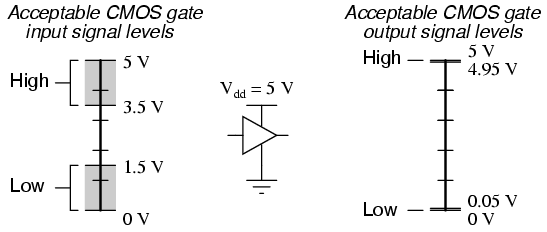It should be obvious from these figures that CMOS gate circuits have far greater noise margins than TTL: 1.45 volts for CMOS low-level and high-level margins, versus a maximum of 0.7 volts for TTL. In other words, CMOS circuits can tolerate over twice the amount of superimposed “noise” voltage on their input lines before signal interpretation errors will result.

CMOS noise margins widen even further with higher operating voltages. Unlike TTL, which is restricted to a power supply voltage of 5 volts, CMOS may be powered by voltages as high as 15 volts (some CMOS circuits as high as 18 volts). Shown here are the acceptable “high” and “low” states, for both input and output, of CMOS integrated circuits operating at 10 volts and 15 volts, respectively:The margins for acceptable “high” and “low” signals may be greater than what is shown in the previous illustrations. What is shown represents “worst-case” input signal performance, based on manufacturer’s specifications. In practice, it may be found that a gate circuit will tolerate “high” signals of considerably less voltage and “low” signals of considerably greater voltage than those specified here.

Conversely, the extremely small output margins shown — guaranteeing output states for “high” and “low” signals to within 0.05 volts of the power supply “rails” — are optimistic. Such “solid” output voltage levels will be true only for conditions of minimum loading. If the gate is sourcing or sinking substantial current to a load, the output voltage will not be able to maintain these optimum levels, due to internal channel resistance of the gate’s final output MOSFETs.

Within the “uncertain” range for any gate input, there will be some point of demarcation dividing the gate’s actual “low” input signal range from its actual “high” input signal range. That is, somewhere between the lowest “high” signal voltage level and the highest “low” signal voltage level guaranteed by the gate manufacturer, there is a threshold voltage at which the gate will actually switch its interpretation of a signal from “low” or “high” or vice versa. For most gate circuits, this unspecified voltage is a single point: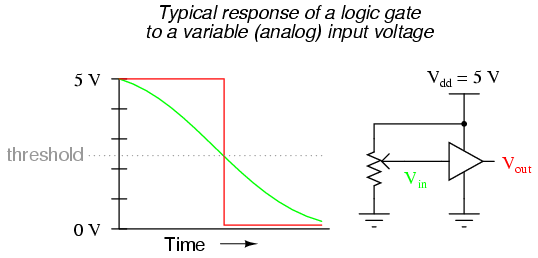In the prsence of AC “noise” voltage superimposed on the DC input signal, a single threshold point at which the gate alters its interpretation of logic level will result in an erratic output: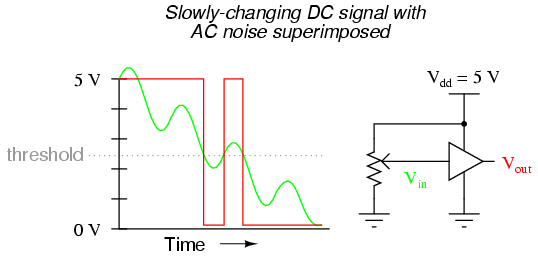If this scenario looks familiar to you, its because you remember a similar problem with (analog) voltage comparator op-amp circuits. With a single threshold point at which an input causes the output to switch between “high” and “low” states, the presence of significant noise will cause erratic changes in the output:The solution to this problem is a bit of positive feedback introduced into the amplifier circuit. With an op-amp, this is done by connecting the output back around to the noninverting (+) input through a resistor. In a gate circuit, this entails redesigning the internal gate circuitry, establishing the feedback inside the gate package rather than through external connections. A gate so designed is called a Schmitt trigger. Schmitt triggers interpret varying input voltages according to two threshold voltages: a positive-going threshold (VT+), and a negative-going threshold (VT-):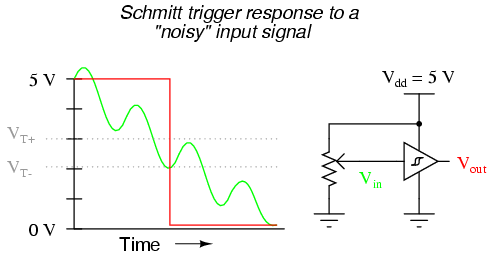Schmitt trigger gates are distinguished in schematic diagrams by the small “hysteresis” symbol drawn within them, reminiscent of the B-H curve for a ferromagnetic material. Hysteresis engendered by positive feedback within the gate circuitry adds an additional level of noise immunity to the gate’s performance. Schmitt trigger gates are frequently used in applications where noise is expected on the input signal line(s), and/or where an erratic output would be very detrimental to system performance.

The differing voltage level requirements of TTL and CMOS technology present problems when the two types of gates are used in the same system. Although operating CMOS gates on the same 5.00 volt power supply voltage required by the TTL gates is no problem, TTL output voltage levels will not be compatible with CMOS input voltage requirements.

Take for instance a TTL NAND gate outputting a signal into the input of a CMOS inverter gate. Both gates are powered by the same 5.00 volt supply (Vcc). If the TTL gate outputs a “low” signal (guaranteed to be between 0 volts and 0.5 volts), it will be properly interpreted by the CMOS gate’s input as a “low” (expecting a voltage between 0 volts and 1.5 volts):However, if the TTL gate outputs a “high” signal (guaranteed to be between 5 volts and 2.7 volts), it might not be properly interpreted by the CMOS gate’s input as a “high” (expecting a voltage between 5 volts and 3.5 volts):Given this mismatch, it is entirely possible for the TTL gate to output a valid “high” signal (valid, that is, according to the standards for TTL) that lies within the “uncertain” range for the CMOS input, and may be (falsely) interpreted as a “low” by the receiving gate. An easy “fix” for this problem is to augment the TTL gate’s “high” signal voltage level by means of a pullup resistor:Something more than this, though, is required to interface a TTL output with a CMOS input, if the receiving CMOS gate is powered by a greater power supply voltage:There will be no problem with the CMOS gate interpreting the TTL gate’s “low” output, of course, but a “high” signal from the TTL gate is another matter entirely. The guaranteed output voltage range of 2.7 volts to 5 volts from the TTL gate output is nowhere near the CMOS gate’s acceptable range of 7 volts to 10 volts for a “high” signal. If we use an open-collector TTL gate instead of a totem-pole output gate, though, a pullup resistor to the 10 volt Vdd supply rail will raise the TTL gate’s “high” output voltage to the full power supply voltage supplying te CMOS gate. Since an open-collector gate can only sink current, not source current, the “high” state voltage level is entirely determined by the power supply to which the pullup resistor is attached, thus neatly solving the mismatch problem: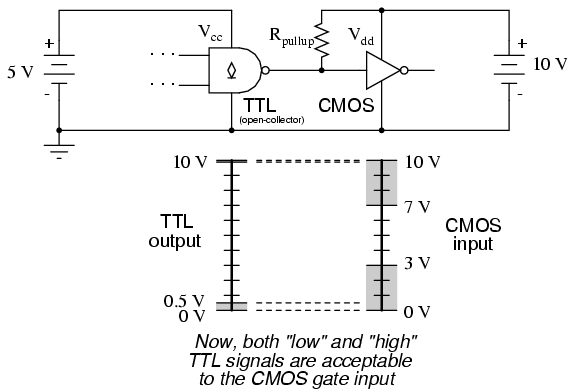Due to the excellent output voltage characteristics of CMOS gates, there is typically no problem connecting a CMOS output to a TTL input. The only significant issue is the current loading presented by the TTL inputs, since the CMOS output must sink current for each of the TTL inputs while in the “low” state.

When the CMOS gate in question is powered by a voltage source in excess of 5 volts (Vcc), though, a problem will result. The “high” output state of the CMOS gate, being greater than 5 volts, will exceed the TTL gate’s acceptable input limits for a “high” signal. A solution to this problem is to create an “open-collector” inverter circuit using a discrete NPN transistor, and use it to interface the two gates together: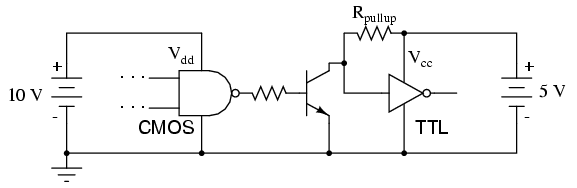The “Rpullup” resistor is optional, since TTL inputs automatically assume a “high” state when left floating, which is what will happen when the CMOS gate output is “low” and the transistor cuts off. Of course, one very important consequence of implementing this solution is the logical inversion created by the transistor: when the CMOS gate outputs a “low” signal, the TTL gate sees a “high” input; and when the CMOS gate outputs a “high” signal, the transistor saturates and the TTL gate sees a “low” input. So long as this inversion is accounted for in the logical scheme of the system, all will be well.

## DIP gate packaging

Digital logic gate circuits are manufactured as integrated circuits: all the constituent transistors and resistors built on a single piece of semiconductor material. The engineer, technician, or hobbyist using small numbers of gates will likely find what he or she needs enclosed in a DIP (Dual Inline Package) housing. DIP-enclosed integrated circuits are available with even numbers of pins, located at 0.100 inch intervals from each other for standard circuit board layout compatibility. Pin counts of 8, 14, 16, 18, and 24 are common for DIP “chips.”

Part numbers given to these DIP packages specify what type of gates are enclosed, and how many. These part numbers are industry standards, meaning that a “74LS02” manufactured by Motorola will be identical in function to a “74LS02” manufactured by Fairchild or by any other manufacturer. Letter codes prepended to the part number are unique to the manufacturer, and are not industry-standard codes. For instance, a SN74LS02 is a quad 2-input TTL NOR gate manufactured by Motorola, while a DM74LS02 is the exact same circuit manufactured by Fairchild.

Logic circuit part numbers beginning with “74” are commercial-grade TTL. If the part number begins with the number “54”, the chip is a military-grade unit: having a greater operating temperature range, and typically more robust in regard to allowable power supply and signal voltage levels. The letters “LS” immediately following the 74/54 prefix indicate “Low-power Schottky” circuitry, using Schottky-barrier diodes and transistors throughout, to decrease power dissipation. Non-Schottky gate circuits consume more power, but are able to operate at higher frequencies due to their faster switching times.

A few of the more common TTL “DIP” circuit packages are shown here for reference: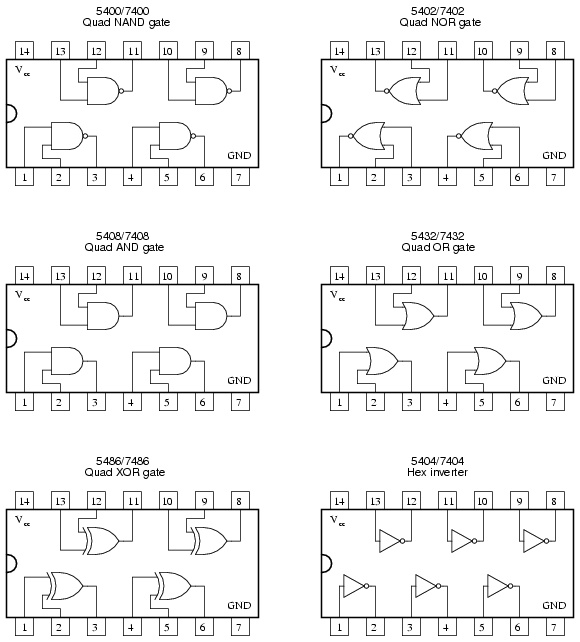Related

COMMENT songs

1. t.b

can we have a visual circuit on a breadboard please of the 1 input NANDA gate